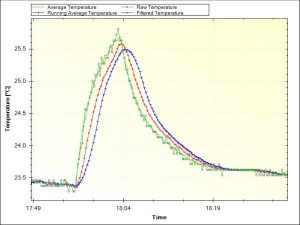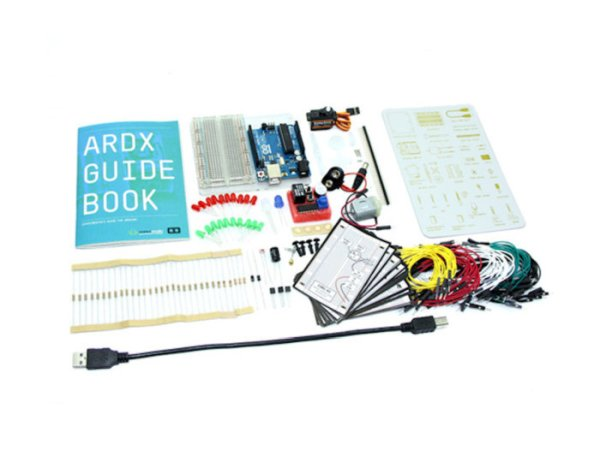# Filtering Noisy Arduino Measurements

Measurements from the real world often contain noise. Loosely speaking, noise is just the part of the signal you didn’t want. Maybe it comes from electrical noise: the random variations you see when calling `analogRead` on a sensor that should be stable. Noise also arises from real effects on the sensor. Vibration from the engine adds noise if you’re measuring the acceleration of a go-kart, for example. Filtering is a method to remove some of the unwanted signal to leave a smoother result.## Filters

We’ll use MegunoLink to compare three different filters:

1. Averaging
2. Running average
3. Expotential filter

MegunoLink’s Time Plot Visualizer will be used to show both the raw, unfiltered, data and the output from the filter. Comparing the raw and filtered on a single plot lets us easily see the effect of the filter. The filters will smooth the data but they can also introduce a lag.

## Something to Filter

To generate some ‘noisy’ data for filtering a thermistor was connected to analog-input 0 on an Arduino Uno. A thermistor is a resistor whose resistance changes with temperature. As temperature increases, resistance goes down; as temperature decreases, resistance goes up. The thermistor was connected in a voltage divider configuration with one leg connected to ground and the other to the analog-input. A 100kΩ resistance was connected between the 3.3V output of the Arduino and the analog-input to provide current for the thermistor.

The temperature measured using a thermistor connected to an Arduino was plotted in MegunoLink. Different filters were compared to see which one smoothed the temperature measurements best.

To measure temperature, the analog value was read. Then the raw analog value was converted to temperature, in degrees Celsius, using the following Arduino code:

After applying the various filters, the data was sent to MegunoLink for plotting:

## Filtering Algorithms

The three filtering algorithms we are going to look at are:

1. Averaging
2. Running average
3. Exponential filter

The screenshot from MegunoLink below shows these algorithms together. A small rise in temperature was created by touching the thermistor to see how the different filters would respond. The grey line is the raw temperature measurement. It is pretty good with not much noise. But there is still room for improvement.

Read more: Filtering Noisy Arduino Measurements

#### This Post / Project can also be found using search terms:

• arduino filtered measure
• megunolinl com/articles/coding/3-methods-filter-noisy-arduino-measurements
• Noise also arises from real effects on the sensor